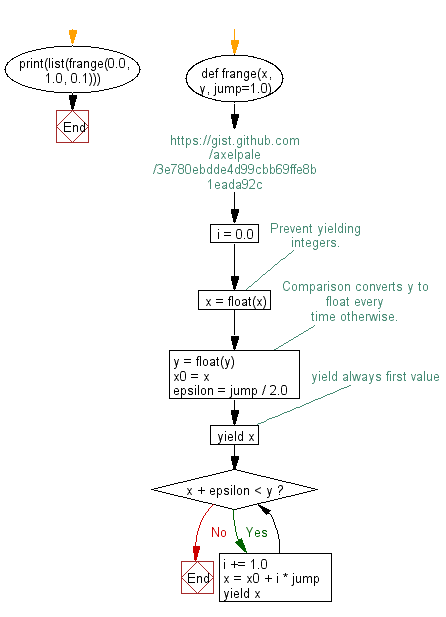﻿ Python Math: Create a range for floating numbers - w3resource# Python Math: Create a range for floating numbers

## Python Math: Exercise-72 with Solution

Write a Python program to create a range for floating numbers.

Sample Solution:-

Python Code:

``````#https://gist.github.com/axelpale/3e780ebdde4d99cbb69ffe8b1eada92c
def frange(x, y, jump=1.0):
i = 0.0
x = float(x)  # Prevent yielding integers.
y = float(y)  # Comparison converts y to float every time otherwise.
x0 = x
epsilon = jump / 2.0
yield x  # yield always first value
while x + epsilon < y:
i += 1.0
x = x0 + i * jump
yield x

print(list(frange(0.0, 1.0, 0.1)))
```
```

Sample Output:

```[0.0, 0.1, 0.2, 0.30000000000000004, 0.4, 0.5, 0.6000000000000001, 0.7000000000000001, 0.8, 0.9, 1.0]
```

Flowchart:## Visualize Python code execution:

The following tool visualize what the computer is doing step-by-step as it executes the said program:

Python Code Editor:

Have another way to solve this solution? Contribute your code (and comments) through Disqus.

What is the difficulty level of this exercise?

Test your Programming skills with w3resource's quiz.

﻿

```>>> students = [{'name': 'John', 'score': 98}, {'name': 'Mike', 'score': 94}, {'name': 'Jennifer', 'score': 99}]